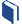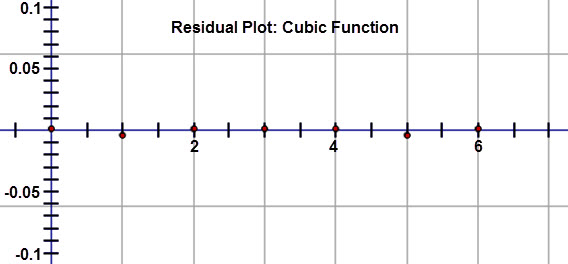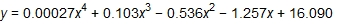# Exploring Other Function Models

###Resources for this lesson:

> Glossary> Calculator Resources> Teacher Resources: Instructional NotesAfter inspecting the residuals, Khalid notices that the residuals are very close to zero and do not show a pattern.  So, a cubic regression is an excellent fit for the data set.

Just to be sure, he decides to calculate a quartic regression.  Here is the quartic regression equation:Khalid: For this regression equation, the leading coefficient is close to 0.  When this happens for a polynomial function, it indicates that the best fit is one degree less.  So, the cubic function is in fact the best fit for the data.